# Machine learning for anomaly detection and condition monitoringThe current article focuses mostly on the technical aspects, and includes all the code needed to set up anomaly detection models based on multivariate statistical analysis and autoencoder neural networks.

My previous article on anomaly detection and condition monitoring has received a lot of feedback. Many of the questions I receive, concern the technical aspects and how to set up the models etc. Due to this, I decided to write a follow-up article covering all the necessary steps in detail, from pre-processing data to building models and visualizing results.

For an introduction to anomaly detection and condition monitoring, I recommend first reading my original article on the topic. This provides the neccesary background information on how machine learning and data driven analytics can be utilized to extract valuable information from sensor data.

The current article focuses mostly on the technical aspects, and includes all the code needed to set up anomaly detection models based on multivariate statistical analysis and autoencoder neural networks.

To replicate the results in the original article, you first need to download the dataset from the NASA Acoustics and Vibration Database. See the downloaded Readme Document for IMS Bearing Data for further information on the experiment and available data.

Each data set consists of individual files that are 1-second vibration signal snapshots recorded at specific intervals. Each file consists of 20.480 points with the sampling rate set at 20 kHz. The file name indicates when the data was collected. Each record (row) in the data file is a data point. Larger intervals of time stamps (showed in file names) indicate resumption of the experiment in the next working day.

#### Import packages and libraries:

The first step is to import some useful packages and libraries for the analysis:

``````# Common imports
import os
import pandas as pd
import numpy as np
from sklearn import preprocessing
import seaborn as sns
sns.set(color_codes=True)
import matplotlib.pyplot as plt
%matplotlib inline

from numpy.random import seed
from tensorflow import set_random_seed

from keras.layers import Input, Dropout
from keras.layers.core import Dense
from keras.models import Model, Sequential, load_model
from keras import regularizers
from keras.models import model_from_json
``````

An assumption is that gear degradation occur gradually over time, so we use one datapoint every 10 minutes in the following analysis. Each 10 minute datapoint is aggregated by using the mean absolute value of the vibration recordings over the 20.480 datapoints in each file. We then merge together everything in a single dataframe.

In the following example, I use the data from the 2nd Gear failure test (see readme document for further info on that experiment).

``````data_dir = '2nd_test'
merged_data = pd.DataFrame()

for filename in os.listdir(data_dir):
print(filename)
dataset_mean_abs = np.array(dataset.abs().mean())
dataset_mean_abs = pd.DataFrame(dataset_mean_abs.reshape(1,4))
dataset_mean_abs.index = [filename]
merged_data = merged_data.append(dataset_mean_abs)

merged_data.columns = ['Bearing 1','Bearing 2','Bearing 3','Bearing 4']
``````

After loading the vibration data, we transform the index to datetime format (using the following convention), and then sort the data by index in chronological order before saving the merged dataset as a .csv file

``````merged_data.index = pd.to_datetime(merged_data.index, format='%Y.%m.%d.%H.%M.%S')
merged_data = merged_data.sort_index()
merged_data.to_csv('merged_dataset_BearingTest_2.csv')
``````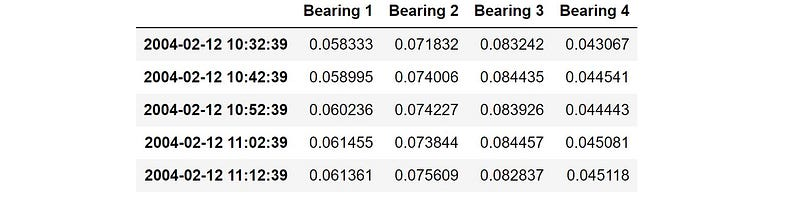Resulting dataframe: “merged_data”

#### Define train/test data:

Before setting up the models, we need to define train/test data. To do this, we perform a simple split where we train on the first part of the dataset (which should represent normal operating conditions), and test on the remaining parts of the dataset leading up to the bearing failure.

``````dataset_train = merged_data['2004-02-12 11:02:39':'2004-02-13 23:52:39']
dataset_test = merged_data['2004-02-13 23:52:39':]
dataset_train.plot(figsize = (12,6))
``````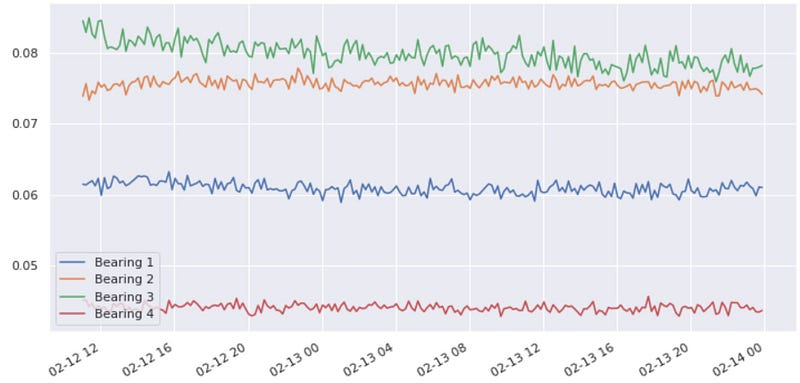Training data: Normal operating conditions

#### Normalize data:

I then use preprocessing tools from Scikit-learn to scale the input variables of the model. The “MinMaxScaler” simply re-scales the data to be in the range [0,1].

``````scaler = preprocessing.MinMaxScaler()

X_train = pd.DataFrame(scaler.fit_transform(dataset_train),
columns=dataset_train.columns,
index=dataset_train.index)
# Random shuffle training data
X_train.sample(frac=1)

X_test = pd.DataFrame(scaler.transform(dataset_test),
columns=dataset_test.columns,
index=dataset_test.index)
``````

### PCA type model for anomaly detection:

As dealing with high dimensional sensor data is often challenging, there are several techniques to reduce the number of variables (dimensionality reduction). One of the main techniques is principal component analysis(PCA). For a more detailed introduction, I refer to my original article on the topic.

As an initial attempt, let us compress the sensor readings down to the two main principal components.

``````from sklearn.decomposition import PCA
pca = PCA(n_components=2, svd_solver= 'full')
X_train_PCA = pca.fit_transform(X_train)
X_train_PCA = pd.DataFrame(X_train_PCA)
X_train_PCA.index = X_train.index

X_test_PCA = pca.transform(X_test)
X_test_PCA = pd.DataFrame(X_test_PCA)
X_test_PCA.index = X_test.index
``````

#### The Mahalanobis distance metric:

The Mahalanobis distance is widely used in cluster analysis and classification techniques. In order to use the Mahalanobis distance to classify a test point as belonging to one of N classes, one first estimates the covariance matrix of each class, usually based on samples known to belong to each class. In our case, as we are only interested in classifying “normal” vs “anomaly”, we use training data that only contains normal operating conditions to calculate the covariance matrix. Then, given a test sample, we compute the Mahalanobis distance to the “normal” class, and classifies the test point as an “anomaly” if the distance is above a certain threshold.

For a more detailed introduction to these technical aspects, you can have a look at my previous article, which covers these topics in more detail.

#### Define functions used in the PCA model:

Calculate the covariance matrix:

``````def cov_matrix(data, verbose=False):
covariance_matrix = np.cov(data, rowvar=False)
if is_pos_def(covariance_matrix):
inv_covariance_matrix = np.linalg.inv(covariance_matrix)
if is_pos_def(inv_covariance_matrix):
return covariance_matrix, inv_covariance_matrix
else:
print("Error: Inverse of Covariance Matrix is not positive definite!")
else:
print("Error: Covariance Matrix is not positive definite!")
``````

Calculate the Mahalanobis distance:

``````def MahalanobisDist(inv_cov_matrix, mean_distr, data, verbose=False):
inv_covariance_matrix = inv_cov_matrix
vars_mean = mean_distr
diff = data - vars_mean
md = []
for i in range(len(diff)):
md.append(np.sqrt(diff[i].dot(inv_covariance_matrix).dot(diff[i])))
return md
``````

Detecting outliers:

``````def MD_detectOutliers(dist, extreme=False, verbose=False):
k = 3. if extreme else 2.
threshold = np.mean(dist) * k
outliers = []
for i in range(len(dist)):
if dist[i] >= threshold:
outliers.append(i)  # index of the outlier
return np.array(outliers)
``````

Calculate threshold value for classifying datapoint as anomaly:

``````def MD_threshold(dist, extreme=False, verbose=False):
k = 3. if extreme else 2.
threshold = np.mean(dist) * k
return threshold
``````

Check if matrix is positive definite:

``````def is_pos_def(A):
if np.allclose(A, A.T):
try:
np.linalg.cholesky(A)
return True
except np.linalg.LinAlgError:
return False
else:
return False
``````

#### Set up PCA model:

Define train/test set from the two main principal components:

``````data_train = np.array(X_train_PCA.values)
data_test = np.array(X_test_PCA.values)
``````

Calculate the covariance matrix and its inverse, based on data in the training set:

``````cov_matrix, inv_cov_matrix  = cov_matrix(data_train)
``````

We also calculate the mean value for the input variables in the training set, as this is used later to calculate the Mahalanobis distance to datapoints in the test set

``````mean_distr = data_train.mean(axis=0)
``````

Using the covariance matrix and its inverse, we can calculate the Mahalanobis distance for the training data defining “normal conditions”, and find the threshold value to flag datapoints as an anomaly. One can then calculate the Mahalanobis distance for the datapoints in the test set, and compare that with the anomaly threshold.

``````dist_test = MahalanobisDist(inv_cov_matrix, mean_distr, data_test, verbose=False)
dist_train = MahalanobisDist(inv_cov_matrix, mean_distr, data_train, verbose=False)
threshold = MD_threshold(dist_train, extreme = True)
``````

#### Threshold value for flagging an anomaly:

The square of the Mahalanobis distance to the centroid of the distribution should follow a χ2 distribution if the assumption of normal distributed input variables is fulfilled. This is also the assumption behind the above calculation of the “threshold value” for flagging an anomaly. As this assumption is not necessarily fulfilled in our case, it is beneficial to visualize the distribution of the Mahalanobis distance to set a good threshold value for flagging anomalies. Again, I refer to my previous article, for a more detailed introduction to these technical aspects.

We start by visualizing the square of the Mahalanobis distance, which should then ideally follow a χ2 distribution.

``````plt.figure()
sns.distplot(np.square(dist_train),
bins = 10,
kde= False);
plt.xlim([0.0,15])
``````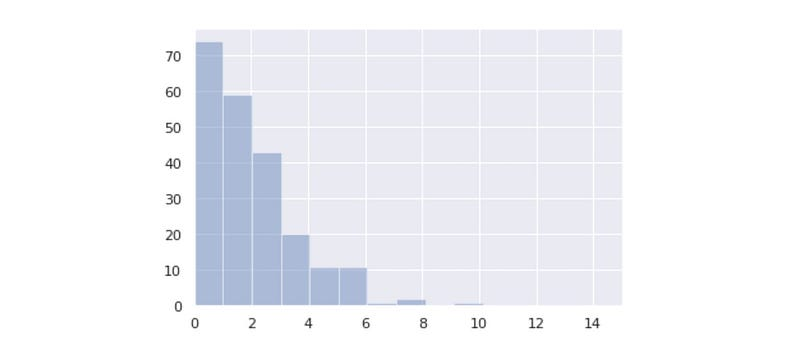Square of the Mahalanobis distance

Then visualize the Mahalanobis distance itself:

``````plt.figure()
sns.distplot(dist_train,
bins = 10,
kde= True,
color = 'green');
plt.xlim([0.0,5])
plt.xlabel('Mahalanobis dist')
``````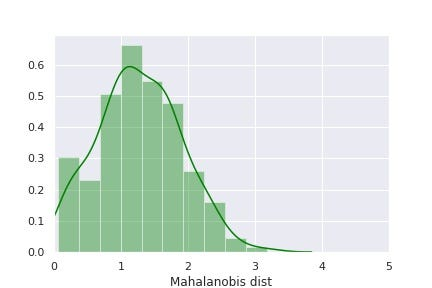From the above distributions, the calculated threshold value of 3.8 for flagging an anomaly seems reasonable (defined as 3 standard deviations from the center of the distribution)

We can then save the Mahalanobis distance, as well as the threshold value and “anomaly flag” variable for the test data in a dataframe:

``````anomaly = pd.DataFrame()
anomaly['Mob dist']= dist_test
anomaly['Thresh'] = threshold
# If Mob dist above threshold: Flag as anomaly
anomaly['Anomaly'] = anomaly['Mob dist'] > anomaly['Thresh']
anomaly.index = X_test_PCA.index
``````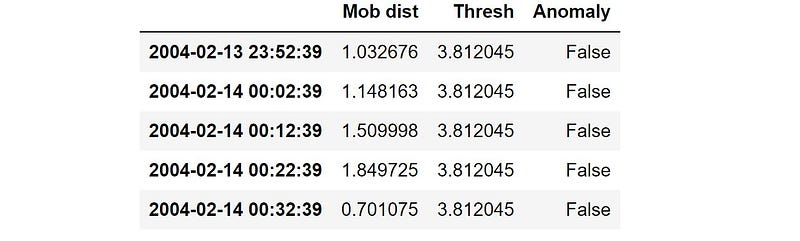Resulting dataframe for the test data

Based on the calculated statistics, any distance above the threshold value will be flagged as an anomaly.

We can now merge the data in a single dataframe and save it as a .csv file:

``````anomaly_alldata = pd.concat([anomaly_train, anomaly])
anomaly_alldata.to_csv('Anomaly_distance.csv')
``````

#### Verifying PCA model on test data:

We can now plot the calculated anomaly metric (Mob dist), and check when it crosses the anomaly threshold (note the logarithmic y-axis).

``````anomaly_alldata.plot(logy=True, figsize = (10,6), ylim = [1e-1,1e3], color = ['green','red'])
``````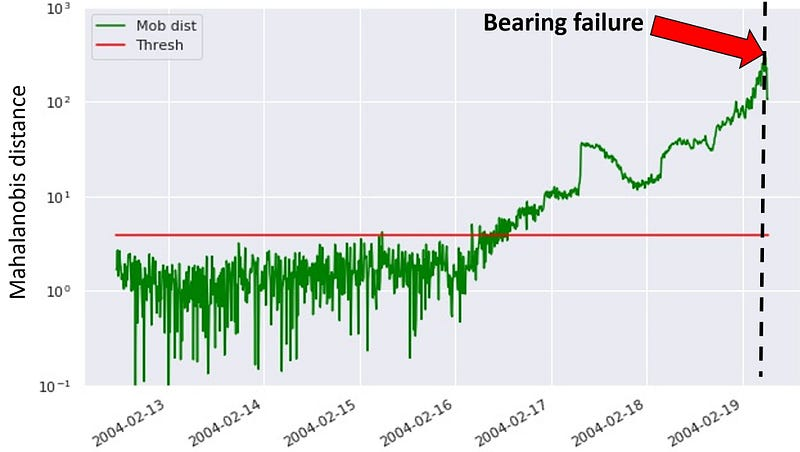From the above figure, we see that the model is able to detect the anomaly approximately 3 days ahead of the actual bearing failure.

### Other approach: Autoencoder model for anomaly detection

The basic idea here is to use an autoencoder neural network to “compress” the sensor readings to a low dimensional representation, which captures the correlations and interactions between the various variables. (Essentially the same principle as the PCA model, but here we also allow for non-linearities among the input variables).

For a more detailed introduction to Autoencoders you can have a look at my previous article, which covers the topic in more detail.

#### Defining the Autoencoder network:

We use a 3 layer neural network: First layer has 10 nodes, middle layer has 2 nodes, and third layer has 10 nodes. We use the mean square error as loss function, and train the model using the “Adam” optimizer.

``````seed(10)
set_random_seed(10)
act_func = 'elu'

# Input layer:
model=Sequential()
# First hidden layer, connected to input vector X.
kernel_initializer='glorot_uniform',
kernel_regularizer=regularizers.l2(0.0),
input_shape=(X_train.shape,)
)
)

kernel_initializer='glorot_uniform'))

kernel_initializer='glorot_uniform'))

kernel_initializer='glorot_uniform'))

# Train model for 100 epochs, batch size of 10:
NUM_EPOCHS=100
BATCH_SIZE=10
``````

#### Fitting the model:

To keep track of the accuracy during training, we use 5% of the training data for validation after each epoch (validation_split = 0.05)

``````history=model.fit(np.array(X_train),np.array(X_train),
batch_size=BATCH_SIZE,
epochs=NUM_EPOCHS,
validation_split=0.05,
verbose = 1)
``````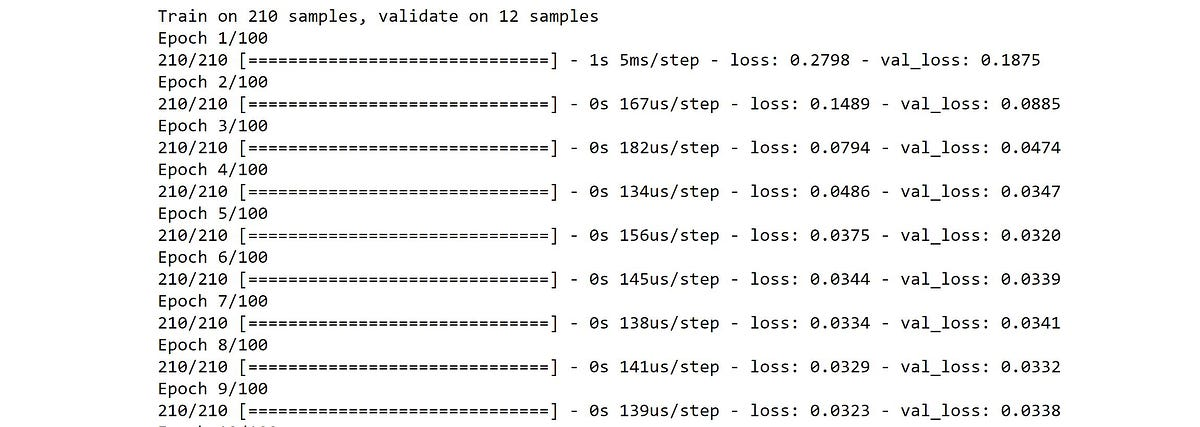Training process

#### Visualize training/validation loss:

``````plt.plot(history.history['loss'],
'b',
label='Training loss')
plt.plot(history.history['val_loss'],
'r',
label='Validation loss')
plt.legend(loc='upper right')
plt.xlabel('Epochs')
plt.ylabel('Loss, [mse]')
plt.ylim([0,.1])
plt.show()
``````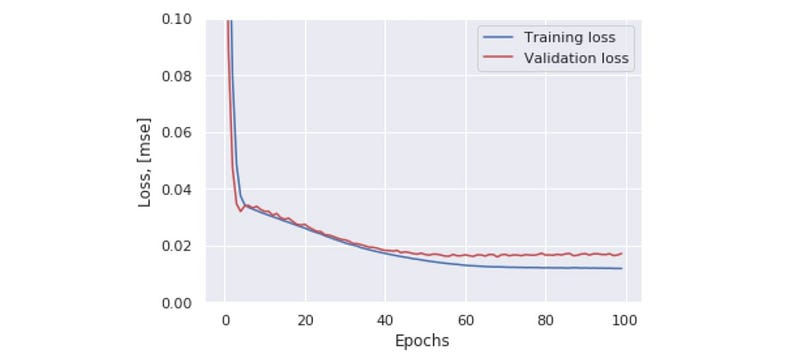Train/validation loss

#### Distribution of loss function in the training set:

By plotting the distribution of the calculated loss in the training set, one can use this to identify a suitable threshold value for identifying an anomaly. In doing this, one can make sure that this threshold is set above the “noise level”, and that any flagged anomalies should be statistically significant above the noise background.

``````X_pred = model.predict(np.array(X_train))
X_pred = pd.DataFrame(X_pred,
columns=X_train.columns)
X_pred.index = X_train.index

scored = pd.DataFrame(index=X_train.index)
scored['Loss_mae'] = np.mean(np.abs(X_pred-X_train), axis = 1)
plt.figure()
sns.distplot(scored['Loss_mae'],
bins = 10,
kde= True,
color = 'blue');
plt.xlim([0.0,.5])
``````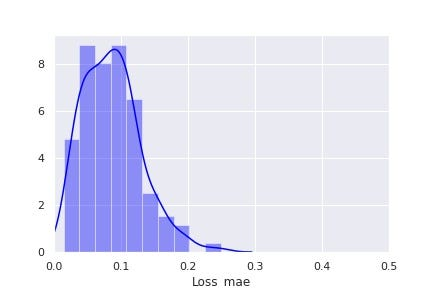Loss distribution, training set

From the above loss distribution, let us try a threshold of 0.3 for flagging an anomaly. We can then calculate the loss in the test set, to check when the output crosses the anomaly threshold.

``````X_pred = model.predict(np.array(X_test))
X_pred = pd.DataFrame(X_pred,
columns=X_test.columns)
X_pred.index = X_test.index

scored = pd.DataFrame(index=X_test.index)
scored['Loss_mae'] = np.mean(np.abs(X_pred-X_test), axis = 1)
scored['Threshold'] = 0.3
scored['Anomaly'] = scored['Loss_mae'] > scored['Threshold']
``````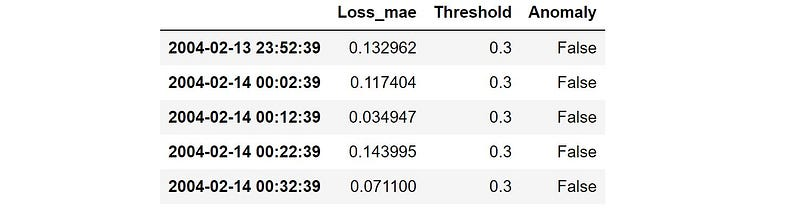We then calculate the same metrics also for the training set, and merge all data in a single dataframe:

``````X_pred_train = model.predict(np.array(X_train))
X_pred_train = pd.DataFrame(X_pred_train,
columns=X_train.columns)
X_pred_train.index = X_train.index

scored_train = pd.DataFrame(index=X_train.index)
scored_train['Loss_mae'] = np.mean(np.abs(X_pred_train-X_train), axis = 1)
scored_train['Threshold'] = 0.3
scored_train['Anomaly'] = scored_train['Loss_mae'] > scored_train['Threshold']
scored = pd.concat([scored_train, scored])
``````

#### Results from Autoencoder model:

Having calculated the loss distribution and the anomaly threshold, we can visualize the model output in the time leading up to the bearing failure:

``````scored.plot(logy=True,  figsize = (10,6), ylim = [1e-2,1e2], color = ['blue','red'])
``````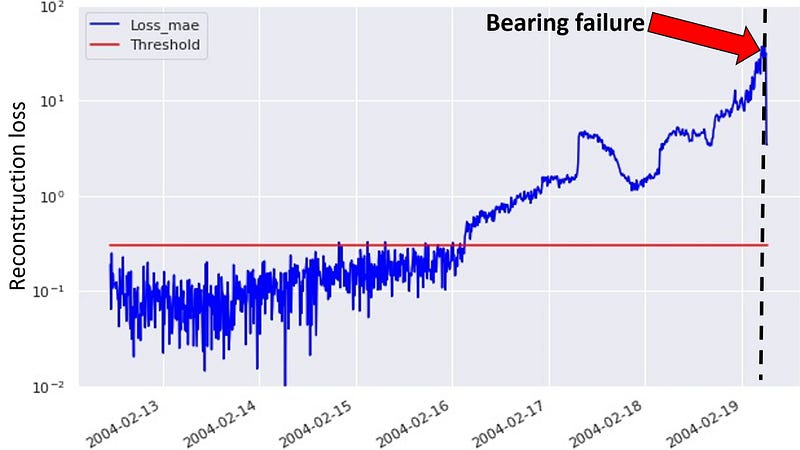### Summary:

Both modeling approaches give similar results, where they are able to flag the upcoming bearing malfunction well in advance of the actual failure. The main difference is essentially how to define a suitable threshold value for flagging anomalies, to avoid to many false positives during normal operating conditions.

I hope this tutorial gave you inspiration to try out these anomaly detection models yourselves. Once you have succesfully set up the models, it is time to start experimenting with model parameters etc. and test the same approach on new datasets. If you come across some interesting use cases, please let me know in the comments below.

Have fun!

## Most popular Data Science and Machine Learning courses — July 2020

Most popular Data Science and Machine Learning courses — August 2020. This list was last updated in August 2020 — and will be updated regularly so as to keep it relevant

## 15 Machine Learning and Data Science Project Ideas with Datasets

Learning is a new fun in the field of Machine Learning and Data Science. In this article, we’ll be discussing 15 machine learning and data science projects.

## Best Free Datasets for Data Science and Machine Learning Projects

This post will help you in finding different websites where you can easily get free Datasets to practice and develop projects in Data Science and Machine Learning.

## 50 Data Science Jobs That Opened Just Last Week

Data Science and Analytics market evolves to adapt to the constantly changing economic and business environments. Our latest survey report suggests that as the overall Data Science and Analytics market evolves to adapt to the constantly changing economic and business environments, data scientists and AI practitioners should be aware of the skills and tools that the broader community is working on. A good grip in these skills will further help data science enthusiasts to get the best jobs that various industries in their data science functions are offering.

## Pipelines in Machine Learning | Data Science | Machine Learning | Python

Machine Learning Pipelines performs a complete workflow with an ordered sequence of the process involved in a Machine Learning task. The Pipelines can also